CATEGORIES:

OXIDATION-REDUCTION REACTIONS

In oxidation-reduction reactions (redox-reactions), the oxidation number of one or more elements in the reacting substances changes. The loosing of electrons by an atom attended by an increase in its oxidation number is called oxidation; the gaining of electrons by an atom attended by a decrease in its oxidation number is called reduction.

A substance containing an element that undergoes oxidation is called a reducing agent. These are almost all metals and some non-metals (C, H2 and others, negatively charged ions of non-metals (S2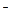, I, N3and others), cations in intermediate oxidation numbers (Sn2+, Fe2+ and others), ions containing elements in intermediate oxidation numbers (SO32, NO2, SnO22and others). In laboratories, such reducing agents as H2, SO2, KI, H3PO3, H2S, HNO2 are usually used.

A substance containing an element that undergoes reduction is called an oxidizing agent. These are atoms and molecules of some non-metals of high activity (O2, O3, Cl2 and others) positively charged metallic ions (Fe3+, Cu2+, Hg2+ and others), particles containing ions in their highest oxidation numbers (MnO4, NO3, SO42, Cr2O72, ClO3and others). The strongest oxidizing agent is electrical current (oxidation on anode). In laboratories, such oxidizing agents as KMnO4, K2Cr2O7, HNO3, H2SO4 (conc.), H2O2, PbO2 are used.

Oxidizing and reducing properties of substances are described with the help of electrode potentials of systems.

The standard electrode potential (jo) is defined as the potential of a given electrode at concentrations (activities) of all the substances participating in the electrode process equal unity.

The dependence of an electrode potential on concentrations of substances participating in electrode processes and on temperature is expressed by the Nernst equation:

j = jo + 2.3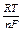log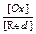where R - the molar gas constant; T - absolute temperature; F - the Faraday’s constant; n - number of electrons participating in the electrode process; [Ox] - concentration of the oxidized form of a substance; [Red] - concentration of the reduced form of a substance.

In case if T = 297 K (25oC),

j = jo +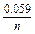log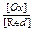The more is the absolute value of redox potential, the stronger are oxidizing properties of the oxidized form.

The possibility of a redox-reaction can be determined from the electromotive force of the reaction (E):

E = j (ox) - j (red)

In case if E > 0, the direct redox-reaction is possible. In case if E < 0, the direct redox-reaction is impossible and the reaction proceeds in the backward direction.

The standard electromotive force E0 is related to the standard Gibbs energy DGof the reaction by the expression

nFE= - DGOn the other hand, DGis related to an equilibrium constant K of a reaction:

DG= - 2.3RT Iog K

It follows then that

nFE= 2.3RT log K

At 25C (298 K), the last equation acquires the form

log K =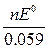To balance redox-reactions, the half-reaction method is used. In acidic media molecules of water and hydrogen-ions enter redox half-reactions. In alkaline media both water molecules and OHions are available. In neutral media the left part of the half-equation contains water molecules and the right part contains either H+ or OHions.

Some examples of redox half-reactions:

Concentrated sulfuric acid

SO42+ 4H+ + 2e = H2SO3 + H2O

SO42+ 8H+ + 6e = S + 4H2O

Nitric acid

NO3+ 4H+ + 3e = NO + 2H2O

NO3+ 3H+ + 2e = HNO2 + H2O

NO3+ 2H+ + e = NO2 + H2O

NO3+ 10H+ + 8e = NH4+ + 3H2O

Manganese compounds

MnO4+ 8H+ + 5e = Mn2+ + 4H2O

MnO4+ 2 H2O + 3e = MnO2 + 4OHMnO4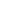+ e = MnO42Chromium compounds

Cr2O72+ 14H+ + 6e = 2Cr3+ + 7H2O

CrO42+ 4H2O + 3e = Cr(OH)63+ 2OHHydrogen peroxide

H2O2 + 2e = 2OHH2O2 + 2H+ + 2e = 2H2O

2H+ + O2 + 2e = H2O2

2H2O + O2 + 2e = H2O2 + 2OHEXPERIMENTAL PART

1. Transfer of an ion to a higher oxidation state

Take 6-8 drops of chromium (III) nitrate and add excess of NaOH. When the precipitate of chromium hydroxide dissolves add 3-4 drops of 3% H2O2 solution. Heat the mixture until the color turns yellow. Write down and balance a corresponding redox-reaction.

2. Redox properties of hydrogen peroxide

a). Take 3 drops of KI solution, add 2 drops of diluted sulfuric acid and 3% H2O2 solution. Add some starch solution to indicate the evaluation of iodine. Write down and balance a corresponding redox-reaction.

b). Take 6 drops of KMnO4 solution, add 2 drops of diluted sulfuric acid and dropwise 3% H2O2. Observe the evaluation of oxygen. Write down and balance a corresponding redox-reaction.

3. Oxidizing properties of potassium permanganate

Fill 3 test tubes with 5 drops of KMnO4 each. Add 2 drops of diluted sulfuric acid into the first test tube, 2 drops of distilled water into the second, and 2 drops of sodium hydroxide into the third one. Add Na2SO3 solution into each test tube until the change of their color. Write down and balance corresponding redox-reactions.

4. Oxidation of cations of d-elements

Take 2 drops of a manganese (II) salt in a test tube, then add 5-6 drops of nitric acid and some crystals of NaBiO3. Observe the appearance of a pink color of HMnO4.. Write down and balance a corresponding redox-reaction.

5. Reducing properties of p-elements

Take 3-4 drops of a solution of SnCl2 in a test tube, add NaOH until the formed precipitate dissolves, and 2-3 of a bithmuth (III) salt solution. Observe the black precipitate of elementary bithmuth. Write down and balance a corresponding redox-reaction.

QUESTIONS AND PROBLEMS

1. Complete the equations of the following reactions and balance them:

(a) K2S + KMnO4 + H2SO4 = S + ....

(b) KI + K2Cr2O7 + H2 SO4 = I2 + ...

( c) K MnO4 + H2O2 = ...

2. Indicate the direction in which the following reactions can proceed spontaneously:

(a) H2O2 + HClO = HCl + O2 + H2O

(b) H3PO4 + 2HI = H3PO3 + I2 + H2O

3. Can a salt of iron (III) be reduced to a salt of iron (II) in an aqueous solution by (a) potassium bromide, (b) potassium iodide?

4. Using the table of standard electrode potentials, calculate the equilibrium constants for the following reactions:

(a) Zn + CuSO4 = Cu + ZnSO4

(b) Sn + Pb(CH3COO)2 = Sn(CH3COO)2 + Pb

Experiment 5

Date: 2015-01-12; view: 926

 <== previous page | next page ==> IONIC PRODUCT OF WATER. PH. HYDROLYSIS OF SALTS | COMPLEX (COORDINATE) COMPOUNDS
doclecture.net - lectures - 2014-2022 year. Copyright infringement or personal data (0.003 sec.)